# C/C++ strings Tutorial

## 1- C-Style String

### 1.1- String

In C++ there are two types of strings, C-style strings, and C++-Style strings.
C-style strings are really arrays, but there are some different functions that are used for strings, like adding to strings, finding the length of strings, and also of checking to see if strings match.
The definition of a string would be anything that contains more than one character strung together. For example, "This" is a string. However, single characters will not be strings, though they can be used as strings.
Strings are arrays of chars. String literals are words surrounded by double quotation marks.
```// Declare a C-Style String.
char mystring[] = { 't', 'h', 'i', 's', ' ', 'i', 's' ,' ', 't', 'e', 'x', 't', '\0'};

// This is a string literal.
char mystring[] = "this is text'';
```
StringLiteralExample.cpp
```#include <stdio.h>

int main() {

// Declare a String literal.
char s1[] = "What is this";

// Print out the string

fflush(stdout);

return 0;
}
```
Running the example: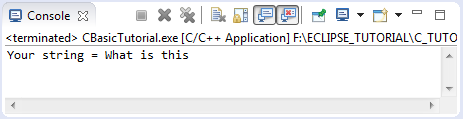• char mystr[] = "what is this";
Above string has 12 characters, to declare that string in C you need to declare an array of characters with 13 elements, remember that the last element in the array is null character (the code is '\ 0'), it means that the end of the string. The last character does not mean in your string, but it is necessary for C programs, such as pointer point to a location of the string it will stand at the first character, and want to retrieve real content of string, program will browse to the next element until it encounters the null character.

### Note:

In case you have an array of characters and  include null character which  is not at the end of the array, or more null characters in the array. But C will only see this array contains a string, including the first character to the first null character in the array.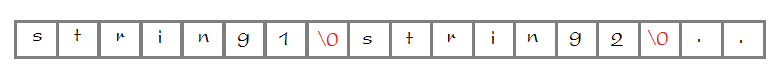The following example declares a character array which has 100  elements used to store the text as user input from the keyboard. In this situation the characters of the input string will be assigned to the first elements of the array and then the null character. And the next element can not be assigned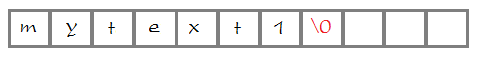StringFromKeyboardExample.cpp
```#include <stdio.h>

int main() {

// Declare an array of characters with 100 elements,
// used to store user input string from the keyboard.
char s1;

fflush(stdout);

// scanf function wait user input from the keyboard.
// (Press enter to end).
// It will scan to get a string and assigned to the variable s1.
// (%s: scan a string does not contain spaces)
scanf("%s", s1);

fflush(stdout);

return 0;
}
```
Running the example: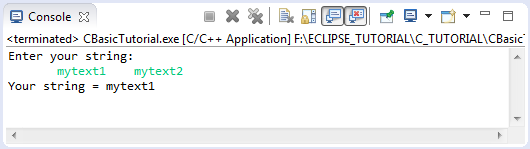### 1.2- Functions for C-style String

C provides several functions to work with String. It is located in the standard <string.h> library . Below I list some common functions of   C relating to string (not all).
Some functions for String:

 Function Name Description size_t strlen(const char *str) Computes the length of the string str up to but not including the terminating null character char *strcpy(char *dest, const char *src) Copies the string pointed to by src to dest.This return a pointer to the destination string dest. int strcmp(const char *str1, const char *str2) Compares the string pointed to by str1 to the string pointed to by str2. If return value > 0 then str1 > str2, else if returns value = 0 then str1 same as str2, else str1 < str2. char *strcat(char *dest, const char *src) Appends the string pointed to by src to the end of the string pointed to by dest.This function return a pointer to the resulting string dest. char *strchr(const char *str, int c) Searches for the first occurrence of the character c (an unsigned char) in the string pointed to by the argument str.
StringFunctionsExample.cpp
```#include <stdio.h>

// Using string library.
#include <string.h>

int main() {

// Declare a string literal.
char s1[] = "This is ";

// Declare a C-Style string
// (With null character at the end).
char s2[] = { 't', 'e', 'x', 't', '\0' };

// Function: size_t strlen(const char *str)
// strlen funtion return length of the string.
// site_t: is unsigned integer data type.
size_t len1 = strlen(s1);
size_t len2 = strlen(s2);

printf("Length of s1 = %d \n", len1);
printf("Length of s2 = %d \n", len2);

// Declare an array with 100 elements.
char mystr;

// Function: char *strcpy(char *dest, const char *src)
// copy s1 to mystr.
strcpy(mystr, s1);

// Function: char *strcat(char *dest, const char *src)
// Using strcat function to concatenate two strings
strcat(mystr, s2);

// Print out content of mystr.

fflush(stdout);

return 0;

}
```
Running the example: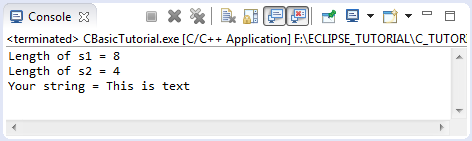## 2- C++ Style String

C++ provides string class which helps you work more easily with the string. The methods which string class provide still support for working with the C-Style string.

### 2.1- Declare string library

You need to declare #include <string> preprocessor directives and declare the use of std namespace.
```// Declare Preprocessor Directives

#include <string>

// Declare to use the namespace std.

using namespace std;
```
Declare a string:
```// Declare a string object.

string mystring = "Hello World";

// If you do not declare using namespace std.
// You must use the full name:

std::string mystring = "Hello World";
```

### 2.2- The methods of the String

Below is list methods of String:

#### 2.2.1- length()

length() is one of the most common methods of string, it returns the string length (number of characters of the string).
LengthDemo.cpp
```#include <iostream>
using namespace std;

int main() {
string str = "This is text";
int len = str.length();

cout << "String Length is : " << len << endl;
return 0;
}
```
Results of running the example: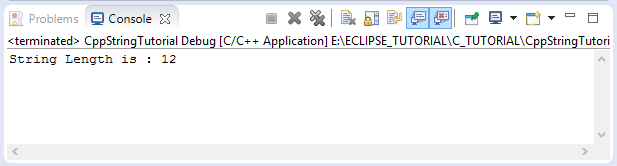#### 2.2.2- append

AppendDemo.cpp
```#include <iostream>
using namespace std;

int main() {

string s1 = "One";
string s2 = "Two";
string s3 = "Three";

cout << "s1 = " + s1 << endl;
cout << "s2 = " + s2 << endl;
cout << "s3 = " + s3 << endl;
cout << " ------------ " << endl;

// Append s2 into s1.
string s = s1.append(s2);

cout << "s1 = " + s1 << endl; // ==> OneTwo
cout << "s = " + s << endl;   // ==> OneTwo

cout << " ------------ " << endl;

// Append s2,s3 into s1.
s = s1.append(s2).append(s3); // ==> OneTwoTwoThree

cout << "s1.append(s2).append(s3) = " + s << endl;

}
```
Results of running the example: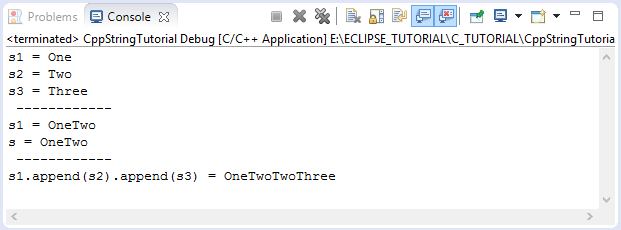#### 2.2.3- find

find is method to find the appear position of a substring  in the current string. This method returns the string::npos constant, if not found.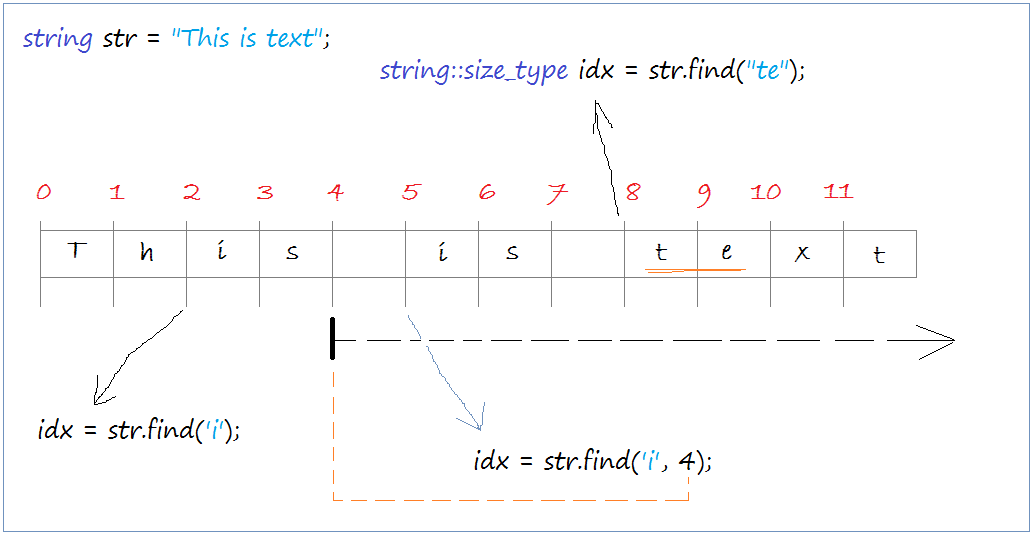FindDemo.cpp
```#include <iostream>
using namespace std;

int main() {

string str = "This is text";
// Find index within this string of the first occurrence 'i'.
// ==> 2
string::size_type idx = str.find('i');

cout << "- find('i') = " << idx << endl;

// Find index within this string of the first occurrence 'i'
// starting the search at index 4.
// ==> 5
idx = str.find('i', 4);
cout << "- find('i',4) = " << idx << endl;

// index within this string of the first occurrence of "te".
// ==> 8
idx = str.find("te");
cout << "- find('te') = " << idx << endl;

}
```
Results of running the example: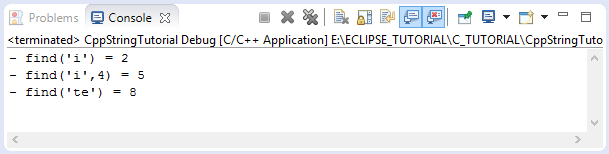#### 2.2.4- substr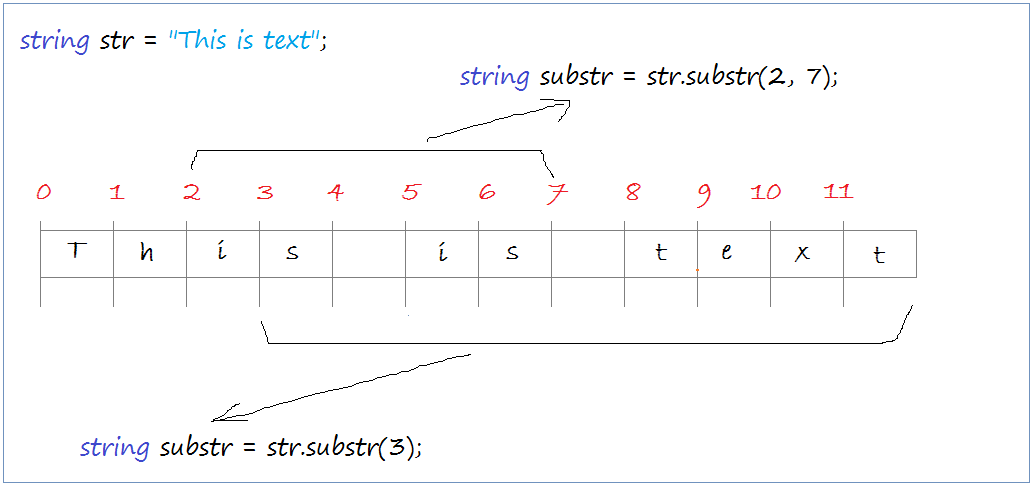SubstrDemo.cpp
```#include <iostream>
using namespace std;

int main() {

string str = "This is text";

// Returns the substring from index 3 to the end of string.
string substr = str.substr(3);

cout << "- str.substr(3)=" << substr << endl;

// Returns the substring from index 2 to index 7.
substr = str.substr(2, 7);

cout << "- str.substr(2, 7) =" << substr << endl;

}
```
Results of running the example: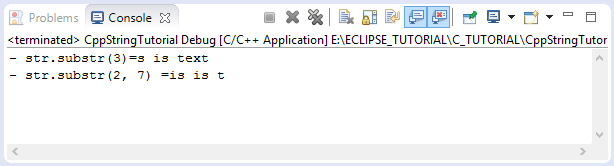#### 2.2.5- replace

Some methods relating to replace.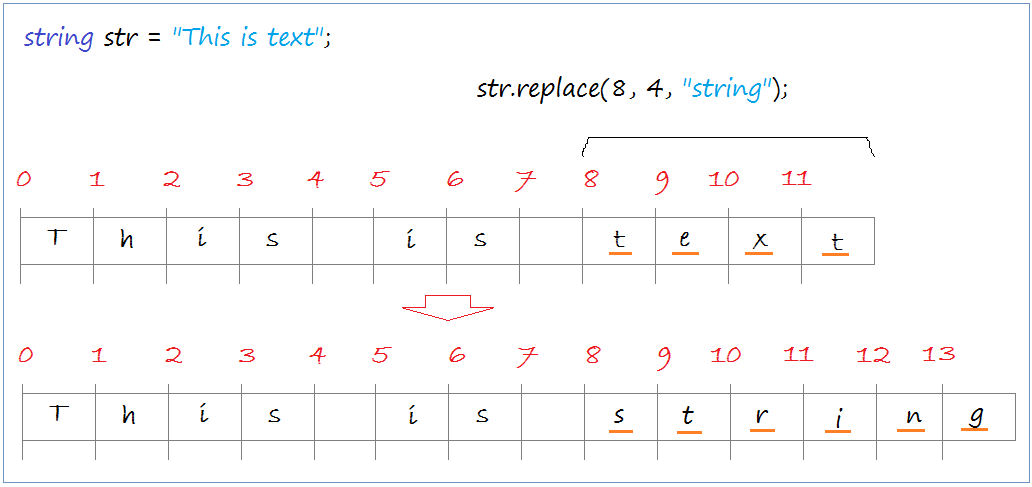ReplaceDemo.cpp
```#include <iostream>
using namespace std;

int main() {

string str = "This is text";

// Replace the 4-character string starting at position 8
// by "string".
string s2 = str.replace(8, 4, "string");

cout << "- str=" << str << endl; // This is string
cout << "- s2=" << s2 << endl;   // This is string
cout << " -------------- " << endl;

// Reset str.
str = "This is text";

// Replace the 4-character string starting at index 8,
// by a substring of another string, 6 characters starting at index 0.
// ==> It is string
string s3 = str.replace(8, 4, "string is important", 0, 6);

cout << "- str=" << str << endl; // It is string
cout << "- s3=" + s3 << endl;    // It is string
cout << " -------------- " << endl;

}
```
Results of running the example: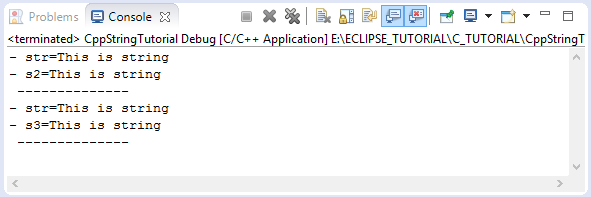An example using the find & replace to replace all the specific substring by new string.
ReplaceAllDemo.cpp
```#include <iostream>
using namespace std;

// A function to find and replace
string replaceAll(string subject, const string& search, const string& replace) {
size_t pos = 0;
while ((pos = subject.find(search, pos)) != string::npos) {
subject.replace(pos, search.length(), replace);
pos += replace.length();
}
return subject;
}

int main() {

string str = "This is text";

cout << "- str: " << str << endl;
cout << " --------------------- " << endl;

// Find "is" and replace by "???".
string result = replaceAll(str,"is", "???");

cout << "- result: " << result << endl;
cout << "- str: " << str << endl;
}
```
Running the example: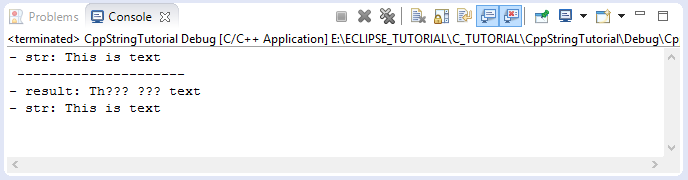#### 2.2.6- insert

InsertDemo.cpp
```#include <iostream>
using namespace std;

int main() {

string str = "This text";

string s2 = str.insert(5, "is ");

cout << "- str=" << str << endl; // This is text
cout << "- s2=" << s2 << endl;   // This is text
cout << " -------------- " << endl;

}
```
Running the example: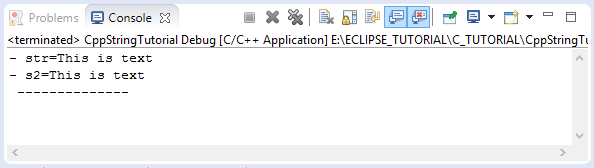#### 2.2.7- upper/lower

Although in string class does not give you a method to convert a string into uppercase or lowercase string, but you can do it with other libraries in C++.
Using transform function of namespace std:
UpperLowerDemo1.cpp
```#include <iostream>
#include <algorithm>
#include <string>

using namespace std;

int main() {

string str = "This is text";

cout << "- str= " << str << endl;

cout << " ----------------"  << endl;

// Convert to uppercase
std::transform(str.begin(), str.end(),str.begin(), ::toupper);

cout << "- str= " << str << endl;

// Reset str
str =  "This is text";

cout << " -------------- " << endl;

// Convert to lowercase.
std::transform(str.begin(), str.end(),str.begin(), ::tolower);

cout << "- str= " << str << endl;
}
```
Running the example: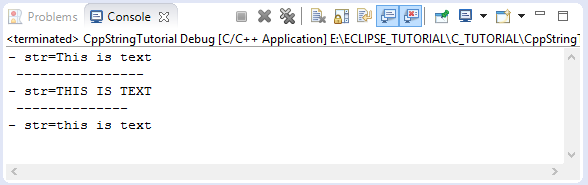#### View more Tutorials:

Maybe you are interested

These are online courses outside the o7planning website that we introduced, which may include free or discounted courses.

•Tic-Tac-Toe Clone - The Complete SFML C++ Game Course
•Byte-Sized-Chunks: Operator Overloading in C++
•Learn C++ Programming Mini Course - Power of Animation
•Concepts of Object Oriented Programming with C++
•C++, 1st program to expert programmer
•Learn the basics of C++ fast! Learn how to Develop Software
•Learn and Understand C++
•Learn C++ Programming The Easy Way
•Learning C++ Memory Management
•*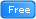* C++, Short and Sweet, Part 1
•How to Program in C++ from Beginner to Professional
•Windows 10 C++ App Development for Startups - C++ Simplified
•C, C++, Java; A Programming MegaPrimer for Beginners
•Learn C++ by Creating
•Start With C / C++ and Object Oriented Programming